# How can I replicate Quantum Break's distortion particle effect?

Quantum Break has this fantastic particle effect, it's a distortion effect like broken glass. I want know how I can replicate this effect? You can see it below, and a full video is available on YouTube: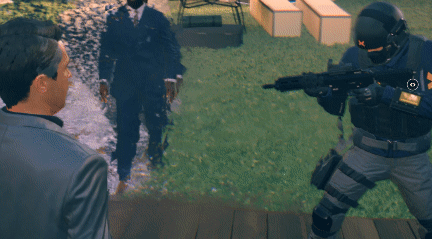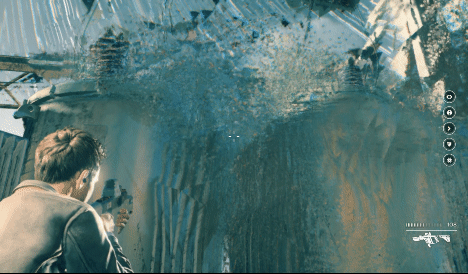# Pyramid Particle

Unity default particle's shape is quad. first you need to change this shape to pyramid by using pyramid object or turns quads to pyramids with a geometry shader.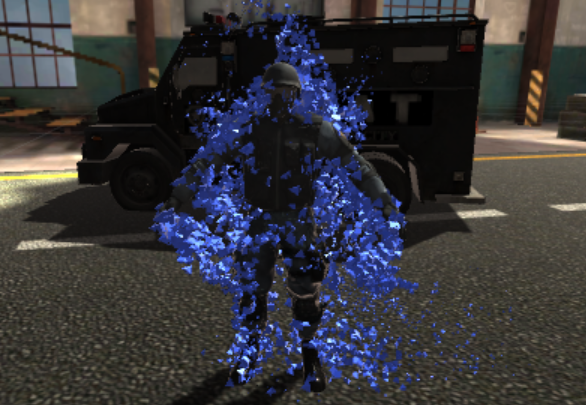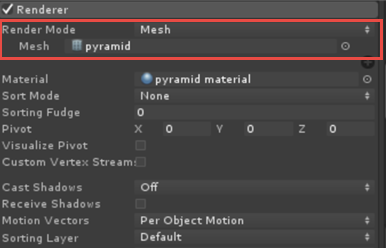# Refraction

To making broken glass effect(Refraction) you can use GrabPass { "TextureName" } that will grab screen contents into a texture.

GrabPass is a special pass type - it grabs the contents of the screen where the object is about to be drawn into a texture. This texture can be used in subsequent passes to do advanced image based effects.

https://docs.unity3d.com/Manual/SL-GrabPass.html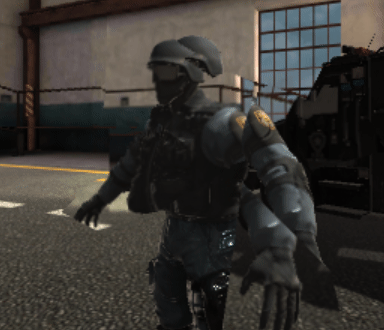Shader "Smkgames/GlassRefraction"
{
Properties{
_Refraction("Refraction",Float) = 0.05
_Alpha("Alpha",Range(0,1)) = 1
}
{
Tags {"Queue"="Transparent" "RenderType"="Transparent"}

Blend SrcAlpha OneMinusSrcAlpha

GrabPass
{
"_GrabTexture"
}

Pass
{
CGPROGRAM
#pragma vertex vert
#pragma fragment frag

#include "UnityCG.cginc"

struct appdata
{
float4 vertex : POSITION;
};

struct v2f
{
float4 grabPos : TEXCOORD0;
float4 vertex : SV_POSITION;
};
sampler2D _MainTex;
float _Alpha,_Refraction;

v2f vert (appdata v)
{
v2f o;
o.vertex = UnityObjectToClipPos(v.vertex);
o.grabPos = ComputeGrabScreenPos(o.vertex);
return o;
}

sampler2D _GrabTexture;

fixed4 frag (v2f i) : SV_Target
{
fixed4 col = tex2Dproj(_GrabTexture, i.grabPos+_Refraction);
return float4(col.rgb,_Alpha);

}
ENDCG
}
}
}


# Using mesh normals

Let’s proceed with a shader that displays mesh normals in world space. I used it because I wanted to look three dimensional broken shape.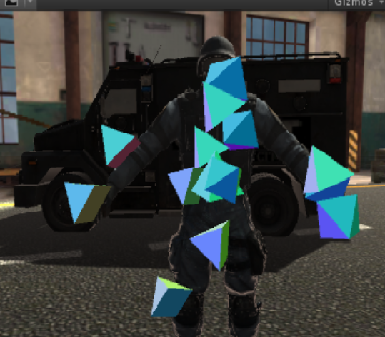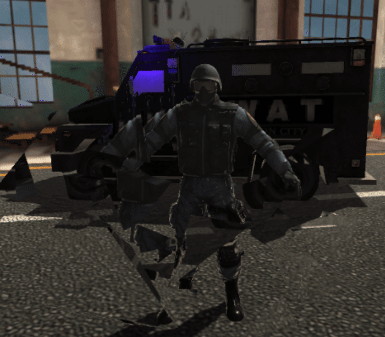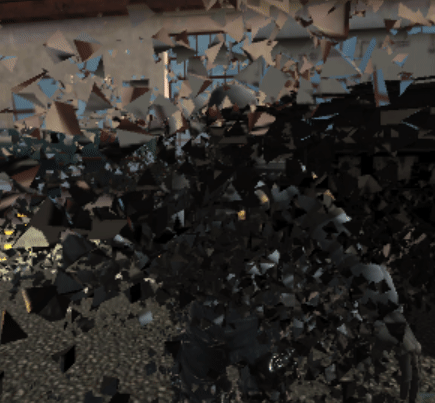Shader "Smkgames/BrokenGlass3D"
{
Properties{
_MainTex("MainTex",2D) = "white"{}
_Alpha("Alpha",Float) = 1
}
{
Tags {"Queue"="Transparent" "RenderType"="Transparent"}
Blend SrcAlpha OneMinusSrcAlpha

GrabPass
{
"_GrabTexture"
}

Pass
{
CGPROGRAM
#pragma vertex vert
#pragma fragment frag

#include "UnityCG.cginc"

struct appdata
{
float4 vertex : POSITION;
float2 grabPos : TEXCOORD0;
float3 normal :NORMAL;
};

struct v2f
{
float4 grabPos : TEXCOORD0;
float4 vertex : SV_POSITION;
half3 worldNormal :TEXCOORD1;

};
sampler2D _MainTex;
float _Intensity,_Alpha;

v2f vert (appdata v)
{
v2f o;
o.vertex = UnityObjectToClipPos(v.vertex);
o.grabPos = ComputeGrabScreenPos(o.vertex);
o.worldNormal = UnityObjectToWorldNormal(v.normal);
return o;
}

sampler2D _GrabTexture;

fixed4 frag (v2f i) : SV_Target
{
fixed4 c = 0;
c.rgb = i.worldNormal*0.5+0.5;
float4 distortion = tex2D(_MainTex,i.grabPos)+_Intensity;
fixed4 col = tex2Dproj(_GrabTexture, i.grabPos+c.r);
return float4(col.rgb,_Alpha);
}
ENDCG
}
}
}


# Heat Distortion

To creating heat distortion you can use flow map

A flow-map is a texture that stores 2d directional information in a texture. The color of the pixel determines what direction it is using the uv-coordinates texture as a base. The more color there is the faster the proportional speed. Example green tells it to go up-left, the center is neutral, and red will go down right. It’s a useful technique for liquid materials like water, and a useful alternative to just a panner node.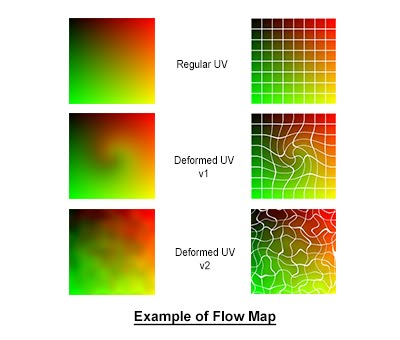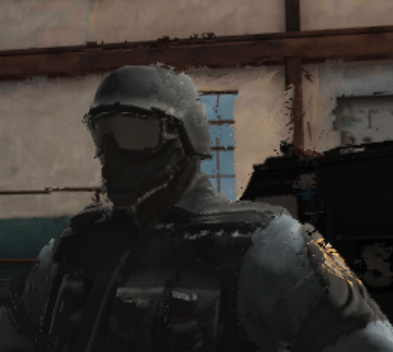Shader "Smkgames/HeatDistortion"
{
Properties{
_DistortionMap("DistortionMap",2D) = "white"{}
_Intensity("Intensity",Float) = 50
_Alpha("Alpha",Range(0,1)) = 1
}
{
Tags {"Queue"="Transparent" "RenderType"="Transparent"}

GrabPass
{
"_GrabTexture"
}

Blend SrcAlpha OneMinusSrcAlpha

Pass
{
CGPROGRAM
#pragma vertex vert
#pragma fragment frag

#include "UnityCG.cginc"

struct appdata
{
float4 vertex : POSITION;
};

struct v2f
{
float4 grabPos : TEXCOORD0;
float4 vertex : SV_POSITION;
};
float _Alpha,_Refraction;

v2f vert (appdata v)
{
v2f o;
o.vertex = UnityObjectToClipPos(v.vertex);
o.grabPos = ComputeGrabScreenPos(o.vertex);
return o;
}

sampler2D _GrabTexture;
float _Intensity;

fixed4 frag (v2f i) : SV_Target
{
float4 distortion = tex2D(_DistortionMap,i.grabPos+_Time.y)+_Intensity;
fixed4 col = tex2Dproj(_GrabTexture, i.grabPos*distortion);

}
ENDCG
}
}
}


another example by using normal: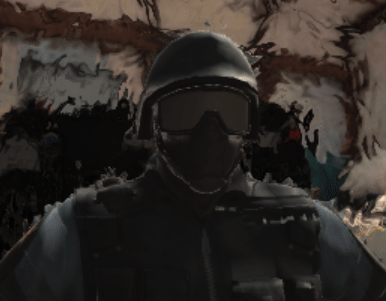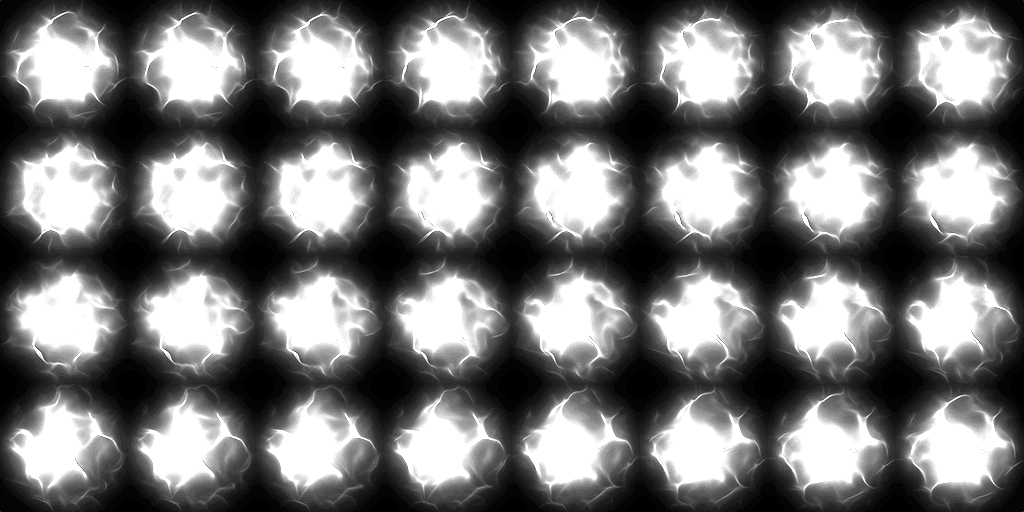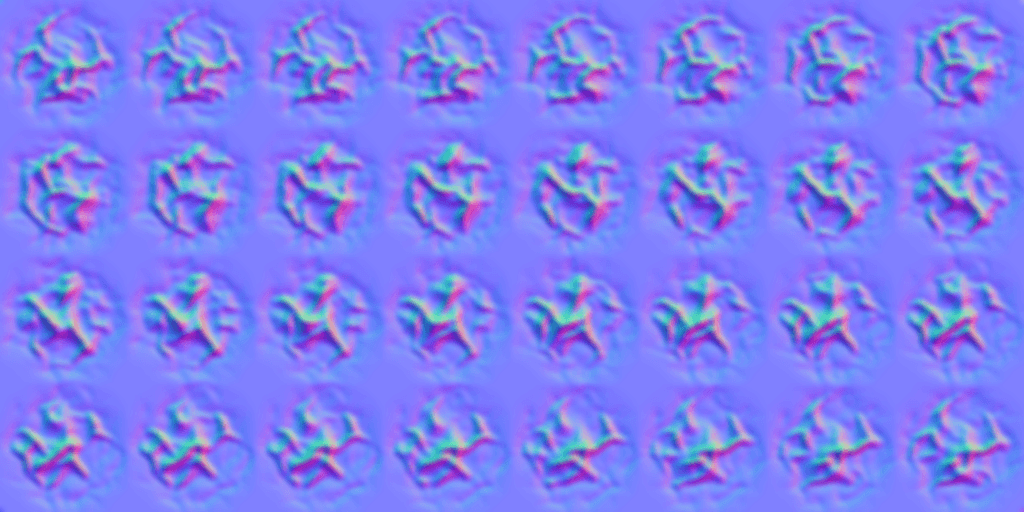Shader "Smkgames/HeatDistortion2" {
Properties {
_CutOut ("CutOut (A)", 2D) = "black" {}
_BumpMap ("Normalmap", 2D) = "bump" {}
_BumpAmt ("Distortion", Float) = 10
}

Category {

Tags { "Queue"="Transparent"  "IgnoreProjector"="True"  "RenderType"="Opaque" }
Blend SrcAlpha OneMinusSrcAlpha
Cull Off
Lighting Off
ZWrite Off
Fog { Mode Off}

GrabPass {
"_GrabTexture"
}
Pass {

CGPROGRAM
#pragma vertex vert
#pragma fragment frag
#pragma fragmentoption ARB_precision_hint_fastest
#pragma multi_compile_particles
#include "UnityCG.cginc"

struct appdata_t {
float4 vertex : POSITION;
float2 texcoord: TEXCOORD0;
};

struct v2f {
float4 vertex : POSITION;
float4 uvgrab : TEXCOORD0;
float2 uvbump : TEXCOORD1;
float2 uvcutout : TEXCOORD2;
};

sampler2D _BumpMap,_CutOut,_GrabTexture;
float _BumpAmt;
float4 _GrabTexture_TexelSize;
float4 _BumpMap_ST,_CutOut_ST;

v2f vert (appdata_t v)
{
v2f o;
o.vertex = UnityObjectToClipPos(v.vertex);
o.uvgrab.xy = (float2(o.vertex.x, o.vertex.y*-1) + o.vertex.w) * 0.5;
o.uvgrab.zw = o.vertex.zw;
o.uvbump = TRANSFORM_TEX( v.texcoord, _BumpMap );
o.uvcutout = TRANSFORM_TEX( v.texcoord, _CutOut );
return o;
}

half4 frag( v2f i ) : COLOR
{
half2 bump = UnpackNormal(tex2D( _BumpMap, i.uvbump )).rg;
float2 offset = bump * _BumpAmt * _GrabTexture_TexelSize.xy;
i.uvgrab.xy = offset * i.uvgrab.z + i.uvgrab.xy;

half4 col = tex2Dproj( _GrabTexture, UNITY_PROJ_COORD(i.uvgrab));
fixed4 cut = tex2D(_CutOut, i.uvcutout);
fixed4 emission = col;
emission.a = (cut.a);
return emission;
}
ENDCG
}
}

}
}


# RGB Split

If you pay attention to your first gif you can see little RGB split.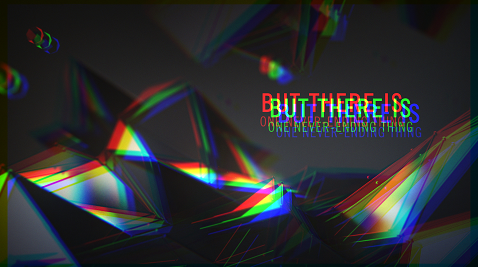Shader "Hidden/RgbSplit"
{
Properties
{
_MainTex ("Texture", 2D) = "white" {}
_NoiseTex1 ("Noise Texture A", 2D) = "white" {}
_NoiseTex2 ("Noise Texture B", 2D) = "white" {}
}
{

Pass
{
CGPROGRAM
#pragma vertex vert
#pragma fragment frag

#include "UnityCG.cginc"

struct appdata
{
float4 vertex : POSITION;
float2 uv : TEXCOORD0;
};

struct v2f
{
float2 uv : TEXCOORD0;
float4 vertex : SV_POSITION;
};

v2f vert (appdata v)
{
v2f o;
o.vertex = UnityObjectToClipPos(v.vertex);
o.uv = v.uv;
return o;
}

sampler2D _MainTex,_NoiseTex1,_NoiseTex2;
float3 colorSplit(float2 uv, float2 s)
{
float3 color;
color.r = tex2D(_MainTex, uv - s).r;
color.g = tex2D(_MainTex, uv    ).g;
color.b = tex2D(_MainTex, uv + s).b;
return color;
}

float2 interlace(float2 uv, float s)
{
uv.x += s * (4.0 * frac((uv.y) / 2.0) - 1.0);
return uv;
}

fixed4 frag (v2f i) : SV_Target
{

float t = _Time.y;

float s = tex2D(_NoiseTex1, float2(t * 0.2, 0.5)).r;

i.uv = interlace(i.uv, s * 0.005);
float r = tex2D(_NoiseTex2, float2(t, 0.0)).x;

float3 color = colorSplit(i.uv, float2(s * 0.02, 0.0));

return float4(color, 1.0);

}
ENDCG
}
}
}